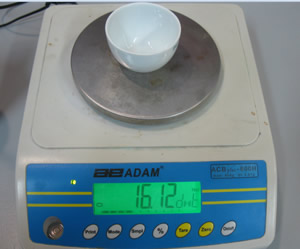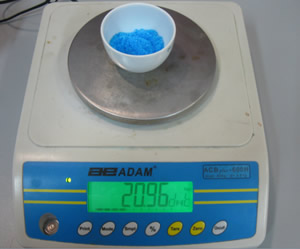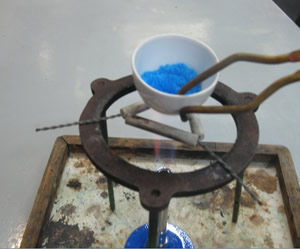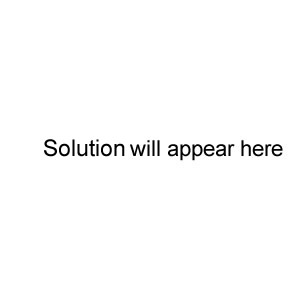Empirical formulae

Copper sulfate is a crystalline solid that has many water molecules embedded in its lattice structure.
The number of water molecules per copper sulfate particle, depends on the conditions the crystals of copper sulfate are in.

The formula of hydrated copper sulfate is CuSO4. XH2O. The number X can vary.
In this activity we will work out X in a given sample of hydrated copper sulfate.

Weigh a crucible as shown on the right.Weigh approximately 5.00 grams of hydrated copper sulfate.Heat the sample over a Bunsen burner. The blue colour will slowly start to turn grey. When all the copper sulfate has turned grey, remove the sample from the flame and allow it to cool for a minute before weighing it.

Record the mass and reheat the sample over a Bunsen flame for a further 5 minutes. Reweigh the sample. Record the mass.
Heat the sample again for a further 5 minutes and repeat until no further mass loss is recorded.Record the final mass of the crucible and anhydrous copper sulfate.Calculations.

1) Fill the table below.

 Item Mass (g) Empty crucible Crucible and hydrated copper sulfate Mass of hydrated copper sulfate Mass of crucible and anhydrous copper sulfate Mass of anhydrous copper sulfate Mass of water removed.

1) Calculate the mass of anhydrous copper sulfate present in your sample.

2) Calculate the amount, in mole, of anhydrous copper sulfate present in your sample. 3) Calculate the mass of water present in the hydrated copper sulfate sample.

4) Calculate the amount, in mole, of water present in your sample.
5) Calculate the simplest mole ratio between copper sulfate and water
6) Calculate XUse the student's results, shown above, to calculate X for this particular sample of hydrated copper sulfate. Solution